# A CONCEPTUAL MULTIDIMENSIONAL MODEL

We now present a simple multidimensional data model "MD" that provides a number of constructs to describe, in an abstract but natural way, the basic notions involved in multidimensional analysis. As is customary in database models, we make a clear distinction between the scheme (which specifies the structure of a concept) and the instance (that is, the actual values associated with a concept).

### Formal Definition of MD

We assume the existence of a finite set of base types such as text, integer, decimal, and date. Each base type t is associated with a domain of base values of that type. We also assume the existence of a countable set of names and a countable set of identifiers (ids). Such ids are values, distinct from base values, that are used to uniquely identify real-life objects.

A dimension has three main components: a set of levels, a set of level descriptions, and a hierarchy over the levels.

Definition 1 [Dimension scheme] An MD dimension scheme D consists of:

• a finite set L of names called levels;

• a finite set Δ of names called level descriptions, for each level in L; each description is associated with a base type t;

• a partial order called roll-up relation on the levels in L; if l1 l2 we say that l1 rolls-up to l2.

There is a natural graphical representation of an MD dimension. Some examples are reported in Figure 2. In this representation, levels are depicted by means of round-cornered boxes and there is a direct arc between the two levels l1 and l2 if l1 l2. Small diamonds depict the descriptions of a level.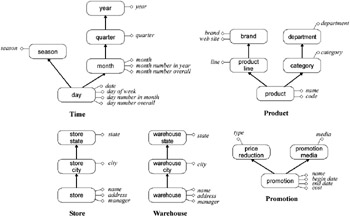Figure 2: Dimension Scheme in the MD Model

Example 3: Figure 2 reports the dimensions for the Toys4All company, as described in Example 1: Time, Product, Store, Promotion, and Warehouse.

As an example, let us consider in more detail the Time dimension. Its levels are day, month, quarter, year, and season. The roll-up relation of Time is the reflexive and transitive closure of the sets of pairs (day, month), (month, quarter), (quarter, year), and (day, season). Thus, for instance, the level day rolls-up to the level month, but also to the level year. Descriptions of the level day are date, day-of-week (mapping each day to the name of the corresponding day), day-number-in-month (mapping each day to the number of the day within its month), and day-number-overall (coding days in consecutive day numbers).

Let us now state precisely what is an instance of a dimension scheme.

Definition 2 [Dimension instance]: An instance of a dimension D=(L, Δ, ) consists of:

• a finite set of (real-world) objects, each of which has a unique id associated with it, for each level l in L, called members of l;

• a function from the members of l to the domain of base type t associated with l, for each level description in Δ;

• a roll-up function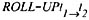from the members of l1 to the members of l2, for each pair of levels l1 and l2 in L such that l1 l2; if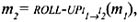we say that m1 rolls-up to m2.

The roll-up functions of a dimension instance must satisfy the following consistency conditions.

Condition 1 [Consistency of roll-up]: The family of roll-up functions of a dimension are consistent if:

1. for each level l, the function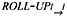is the identity on the members of l; and

2. if a level l1 rolls-up to l2 in different ways (e.g., rolling-up through either l' or l“), then the members of l1 roll-up to elements of l2 in a consistent way, that is: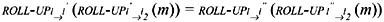for each member m of l1.

Note that, as is customary in conceptual models, a member of a dimension level is not a value but is the object itself (e.g., a member of the store level is the actual building, not its name and address). In fact, although this object has an id and a number of values (the descriptions) associated with it, its existence and identity are clearly independent of them.

We are now ready to introduce the general notion of multidimensional database scheme. This has two main components: a collection of dimensions and a number of data cube schemes, which are defined over levels of the dimensions.

Definition 3 [Multidimensional Scheme]: A multidimensional scheme consists of:

• a finite set D of dimension schemes;

• a finite set F of data cube schemes of the form: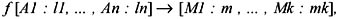where f is a name, each Ai (1 i n) is a distinct name called attribute of f, each li is a level of D, each Mj (1 j k) is a distinct name called measure of f, and each mj is either a base type or a level of D.

Note that in MD there is a uniform treatment of measures and dimensions, as a measure can be not only a simple value but also a level of a dimension. This allows the analyst to transform measures into attributes and vice versa (Cabibbo & Torlone, 1998b), an important functionality that any OLAP system should have (Pedersen, 2000).

Data cube schemes can also be naturally represented by means of diagrams. An example that refers to the dimensions in Figure 2 is given in Figure 3: facts are represented by boxes and measures by circles.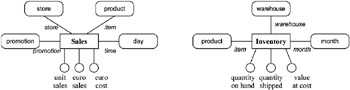Figure 3: Two Data Cube Schemes Over the Dimensions in Figure 2

Example 4: A multidimensional scheme for the business processes of the Toys4All Company described in Example 1: and Example 2: can be defined using the dimension schemes of Example 3: Specifically, two data cubes, Sales and Inventory, can be used to model the sale process and the warehouse process respectively. The schemes of these data cubes are represented graphically in Figure 3.

The data cube Sales describes daily sales, detailed by item, store, and promotion. Its attributes are time (at the day level of the time dimension, describing the day in which the sale occurred), item (the product sold), store (the store having sold the product), and promotion (the promotion applied to the sale). Its measures are unit-sales (the number of items sold), euro-sales (the income of the sale, in Euros), and euro-cost (the cost price of the items sold).

The data cube Inventory is instead used to represent the inventory levels of the various products, detailed by warehouse and month. Specifically, inventory levels are measured at the end of each month. The measures of this data cube are quantity-on-hand (the quantity in stock of a product at the end of the month), quantity-shipped (the quantity shipped from the warehouse during the month), and value-at-cost (the value of the quantity in stock, at cost price).

Before introducing the notion of instance of a data cube scheme, two preliminary notions are needed.

Let D = (D,F) be a multidimensional scheme, f[A1 : l1,, An : ln] [M1 : m, , Mk : mk] be a data cube scheme in F, and d be an instance of D.

Definition 4 [Conceptual coordinate]: A(conceptual) coordinate for f over d is a tuple over the attributes of f, that is, a function mapping each attribute Ai to a member of the level li occurring in d.

Definition 5 [Fact]: A fact for f over d is a tuple over the measures of f, that is, a function mapping each measure name Mj to either a value (if mj is a base type) or a member in d (if mj is a level).

We are now ready to introduce the notion of instance of a multidimensional scheme.

Definition 6 [Instance of multidimensional scheme]: An instance of a multidimensional database scheme (D,F) is composed of:

• a dimension instance d for each dimension scheme in D;

• a partial function called data cube mapping coordinates for f over d to facts for f over d, for each data cube scheme f in F.

An entry of a data cube c is a coordinate over which the instance of c is defined.

Example 3: A possible instance for the multidimensional scheme of Example 4: is shown in Figure 4. In this example, level members are represented by their ids.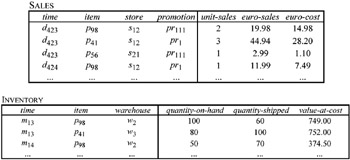Figure 4: A Sample Instance Over the Multidimensional Scheme of Example 4:

A coordinate over the data cube scheme Sales is, for example,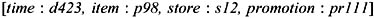where d423 is, for instance, the id associated with the physical item at hand.

The actual instance associates with this entry the value 2 for the measure unit-sales, the value 19.98 for the measure euro-sales, and the value 14.98 for the measure euro-cost.

In Figure 4, data cubes are graphically represented as a table. This representation suggests how data cubes can be implemented using the relational model: a data cube over a scheme f can be represented by a relation over the attributes of f, with additional columns for the measures. The attributes of f form the key of the relation. In practice, a data cube having n attributes and m measures can also be represented by means of an n-dimensional array in which each (non-null) entry corresponds to an entry of f and is associated with an m-tuple of measures. This representation recalls the way in which multidimensional systems usually store data, thus confirming that the MD is a conceptual model which describes multidimensional data independently of any specific (logical) implementation.

It is apparent that the notation we have used for coordinates resembles subscripting into a multi-dimensional array (although in a non-positional way). However, there is an important difference between data cubes and multi-dimensional arrays. Specifically, in arrays, "physical" coordinates vary over intervals within (linearly ordered) domains of values, whereas domains over which coordinates range in the MD model are conceptual entities. In this sense, our notion of coordinate is "conceptual."

Roll-up functions are a distinctive feature of the model proposed: they describe intentionally how members of different levels are related. This description is independent of any effective implementation: roll-up functions can be implemented by means of materialized relations, built-in functions, or external procedures. Moreover, roll-up functions provide a powerful tool for querying multidimensional data, as they can be used to specify how data can be grouped, and how data cubes involving data at different levels of granularity can be joined (Cabibbo & Torlone, 1997, 1998b).

### Basic Features of a Multidimensional Model

The MD data model presented in the previous section exhibits those fundamental features that any multidimensional model should include in some form in order to be suitable for OLAP applications. According to Pederson (2000) and Blaschka et al. (1998), these "mandatory" features can be summarized as follows.

• Explicit separation of structure and contents. This is indeed a basic requirement of database models that make a clear distinction between the schema, which describes the structure of data, and the instances, which correspond to the actual contents.

• Explicit notions of dimension and data cube. These are the basic concepts of multidimensional data representation, as we discussed earlier.

• Explicit hierarchies in dimensions. A dimension should be structured into a hierarchy of levels to suggest the modalities in which data can be grouped along dimensions.

• Multiple hierarchies in each dimension. In one dimension, there can be more than one path along which to aggregate data. This is captured in MD by having a partial order relationship between the levels of a dimension.

• Level attributes. Other descriptive properties of the analysis dimensions, independent of the hierarchy relationship among levels, should also be representable. Level descriptions are used in MD for this purpose.

• Measure sets. This refers to the possibility of defining complex cell structures (grouping more than one measure) related to the same fact. In MD this is implemented by associating several measures to the same cube coordinate.

• Symmetrical treatment of dimensions and measures. The data model should allow measures to be treated as dimensions and vice versa. This is important because there are concepts (for instance, the age of customers) that can be measured (for instance, the average age of customers can be of interest) but which can also be used to group facts. This aspect is implemented in MD by allowing measures to be defined over dimension levels. This solution also makes it possible to register factual data at different granularities.

### Advanced Features of a Multidimensional Model

There are a number of further advisable features that a conceptual multidimensional model should support. We have classified these features as "advanced" because they model concepts that either: i) are difficult to represent in a simple way (such as the notion of "summarizability"), or ii) serve to capture specific application cases. Adopting once more a terminology inherited from Pedersen (2000) and Blaschka et al. (1998), these basic features can be summarized as follows.

• Support for aggregation semantics. The data model should provide a support for the identification of aggregations whose result is incorrect, that is, meaningless to the user. This undesirable situation may occur for two main reasons.

• – A single fact can be counted more than once. Let us consider for instance the data cube Sales of our case study, whose scheme is described in Example 4: and reported in Figure 3. If we need the number of sales with respect to a specific media used for their promotion, we should only count a given sale once, even if several promotions have been applied to the sale.

• – Some types of aggregation along certain paths of a dimension can be meaningless for a specific type of measure. For example, it may not be meaningful to add inventory levels of different products together, but calculating their average may make sense. This concept is strictly related to the notion of summarizability studied in the context of statistical databases (Lenz & Shoshani, 1997; Rafanelli & Shoshani, 1990), whichdefines when an aggregation, for instance, total sales, can be calculated by directly combining results from lower-level aggregations, for instance, the sales for each store. This problem has been recently investigated by various authors (Hurtado & Mendelzon, 2001; Lehner, Albrecht, & Wedekind, 1998).

• Support for non-standard aggregations of facts. There are various possible cases.

• Non-strict hierarchies. The hierarchy of levels in a dimension is non-strict if some of the mappings between the members of one level to the members of a higher level are many-to-many rather than one-to-many relationships. In our example, the Product dimension, described in Example 1 and represented in Figure 2, becomes non-strict if, for instance, a product can be classified according to different categories. The MD model can be extended to include non-strict hierarchies by assuming that the mappings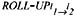are simple binary relations over members of levels l1 and l2 such that l1 rolls-up to l2, rather than functions.

• Non-onto hierarchies. A hierarchy in a dimension is "onto" if, for each member m of a level, there is a member m' of a lower level (if any) such that m' rolls-up to m. This property is not satisfied in our case study if, for example, there is a brand in an instance of the Product dimension (see Figure 2) with no associated product. In MD non-onto hierarchies are allowed as no restrictions are posed on the functions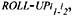which can be therefore non-onto.

• Non-covering hierarchies. A hierarchy in a dimension is non-covering if the member of a level rolls-up to a member of a higher level in the hierarchy by "skipping" one or more intermediate levels. In the Toys4All example, this may happen if, for example, in an instance of the Store dimension (see again Figure 2), there is a member of the Store level that rolls-up to a member of the State level, without rolling-up to any members of the City level. This would occur if the corresponding store is located not in a city but in a ruralarea. In MD non-covering hierarchies can be supported by allowing the roll-up functions to be partial.

• Many-to-many relationships between facts and dimensions. It may happen that the relationship between a fact and its corresponding dimensions is not a many-to-one mapping. In our case study, it may be the case that a specific sale (a row in the fact cube reported in Figure 4) is actually associated with a combination of promotions rather than just one. This is not strictly forbidden in the model (new rows can be added for this purpose) but can lead to incorrect aggregations (see above). This problem can be solved in many cases with an appropriate instantiation of the dimensions (Pedersen, Jensen, & Dyreson, 2001).

• Handling change and time. Schemes and data change over time, and there may sometimes be an interest in performing analysis across changes. In our example, a category of products might be moved from one department to another; we would then analyze the impact of this change on the number of sales. The problem of the management of slowly changing dimensions (Kimball, 1996) is related to this aspect. The maintenance of data cubes under dimension updates is also a relevant problem and has been recently investigated (Hurtado, Mendelzon, & Vaisman, 1999). Temporal analysis can also be of interest, for instance, the variations in inventory levels over time. Approaches taken in temporal data models (Tansel et al., 1993) could be applied to deal with these cases.

• Handling imprecision. Any real application must deal with the intrinsic problem of imprecision in representing and managing information. This problem has been widely studied in conceptual modeling. However, few studies have addressed this interesting and important problem in the context of multidimensional analysis, where imprecise data (for instance, the presence of missing values) can lead to incorrect results in calculating aggregations (Dyreson, 1996; Pedersen, Jensen, & Dyreson, 1999). A simple way to include a notion of imprecision in the measurement of facts in MD is to allow the presence of null values in data cubes. Conversely, incomplete knowledge of the dimension hierarchies can be taken into account by assuming that the roll-up functions are partial.Multidimensional Databases: Problems and Solutions
ISBN: 1591400538
EAN: 2147483647
Year: 2003
Pages: 150